# Everything About the XGBoost Classifier

By Vineeth Kumar Category Machine Learning Reading time 5-6 mins Published on Feb 04, 2022

What is XGBoost Machine Learning Algorithm that's taking over the world?

There was a time when regression modeling was all that an analyst must remember for predictive analysis. Knowledge of linear and logistic regression was enough to build a statistical model based on regression. However, times changed, and with the new technological upgradation, one of the most advanced approaches to solving prediction problems is now XGBoost or Extreme Gradient Boosting.

XGBoost Classifier is a well-known gradient-boosting toolkit used for GPU programming, distributed systems, and parallel processing. XGBoost algorithm has been at the forefront of many well-known industry applications.

We'll look into the XGBoost machine learning explained in detail below.

## What is the XGBoost Classifier?

XGBoost classifier is a boosting machine learning algorithm applied for structured and tabular data. It implements gradient-boosted decision trees designed for speed and performance. Furthermore, It is an extreme gradient boost algorithm, meaning it's a machine learning algorithm with a wide range of applications. The applications include solving problems regarding regression, classification, etc.

## How does XGBoost work?

XGBoost works with small to medium-sized tabular datasets and is an ensemble modeling technique. Prediction problems with large unstructured datasets can be handled by Artificial Neural Networks (ANN) algorithms or gradient-boosting machines. However, XGBoost algorithms are capable of handling small datasets with speed and accuracy.

### So, What is Ensemble Modeling?

XGBoost machine learning classifier is an ensemble learning method. It is not always sufficient to depend solely on the outcomes of a single machine learning model. Through ensemble learning, we can systematically merge the predictive ability of several learners. The resultant is a single model that aggregates output from several models.

The models that form the ensemble, also known as base learners, could be either from the same or different learning algorithms.

For example, bagging and boosting are two widely used ensemble learners. Decision trees have central usage for bagging and boosting among several other statistical models.

#### Ensemble Algorithms

In machine learning and predictive modeling, ensemble learning mixes numerous learners (models) to increase overall performance. Simultaneously, it improves predictiveness and accuracy. Ensemble models are powerful because they can integrate thousands of tiny learners skilled in underlying data subsets.

Some intriguing conclusions to be made from these are:-

• Bagging greatly reduces the basic model's variance.

• Boosting reduces the bias as well.

• Stacking also enhances overall predictive power.

#### Ensemble Modeling Types

Considering how the sub-learners are created, we can classify ensemble methods into two groups:

1. When learners are developed sequentially (AdaBoost vs XGBoost): It relies on the interdependence of basic learners. Each learner impacts the next and may determine a regular behavior pattern. Here, AdaBoost becomes a well-known example of such a sequential ensemble method.

2. When learners are developed parallelly (XGBoost vs Random Forest): The base learners are constructed individually to study and utilize the impacts of their independence and decrease the error by combining the findings. Random forest is one example of this method in action.

## Unique features of the XGBoost Classifier

XGBoost is a popular implementation of gradient boosting. Let's go through some of the qualities of XGBoost.

### Regularization

XGBoost machine learning classifier can penalize complex models through L1 and L2 regularization. In addition, regularization helps in preventing overfitting.

### Solving sparse data

Data become sparse due to incomplete information processing operations such as one-hot encoding. Therefore, XGBoost incorporates a sparsity-aware split-finding algorithm to handle different types of sparsity patterns in the data.

### Weighted quantile sketch

Most existing tree-based algorithms can find the split points when the data points are of equal weight (using a quantile sketch algorithm). However, XGBoost has a distributed weighted quantile sketch algorithm to handle weighted data effectively.

### Block structure for parallel learning

The XGBoost machine learning classifier can use multiple cores on the CPU for faster computing. This is possible because of a block structure in its system design. Data in such memory units are called blocks.

Unlike other machine learning algorithms, XGBoost enables the data layout to be reused by subsequent iterations instead of computing it again. This feature is useful for steps like split finding and column sub-sampling.

### Cache awareness

The XGBoost classifier requires non-continuous memory access to get the gradient statistics by row index. Hence, XGBoost Classifier has been designed to make optimal use of hardware. This is done by allocating internal buffers in each thread, where one can store the gradient statistics.

### Out-of-core computing

This functionality optimizes and maximizes the accessible disc space when dealing with large datasets that cannot fit into memory.

## Solving the XGBoost mathematically:-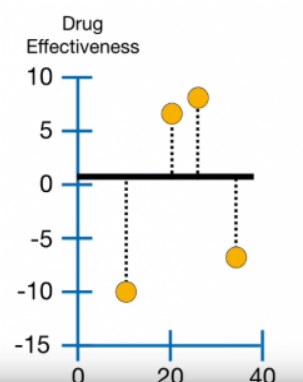This is basic training data, showing drug dosage on the x-axis and drug effectiveness on the y-axis. The above two observations (6.5, 7.5) have a large value for drug effectiveness, which means that the drug was helpful, and the below two observations (-10.5, -7.5) have a relatively low value for drug effectiveness that the drug was harmful.

The very first step in fitting the XGBoost machine learning classifier to the training data is to make an initial prediction. It is 0.5 if the XGBoost classifier is used for regression or classification.

The broad black horizontal line corresponds to the forecast of 0.5.

Unlike extreme Gradient Boost, which typically uses regular off-the-shelf, regression trees, XGBoost machine learning uses a unique regression tree called an XGBoost tree.

### Computing the Residuals' Quality or Similarity scores:-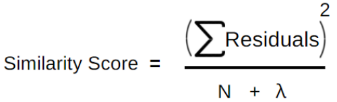Here, N is the number of residuals

and, λ is a regularization parameter

So we split the observations into two groups based on whether or not the Dosage<15.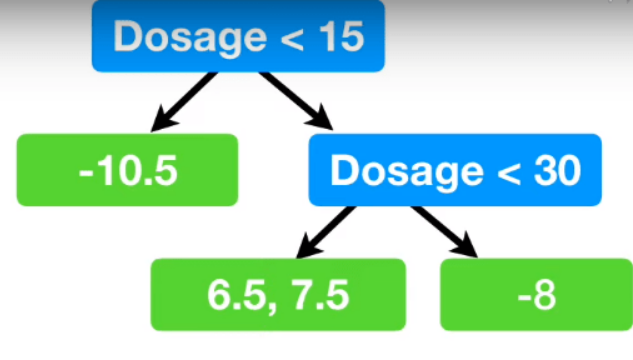The observation on the left is the only one with a Dosage<15. All of the other residuals go to the leaf on the right.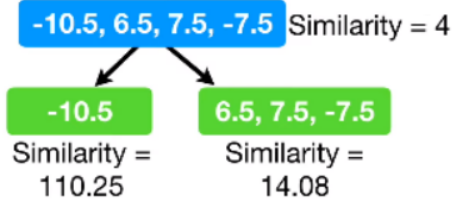When we calculate the similarity score for the observations -10.5, -7.5, 6.5, 7.5, while putting λ = 0.

We got similarity = 4 and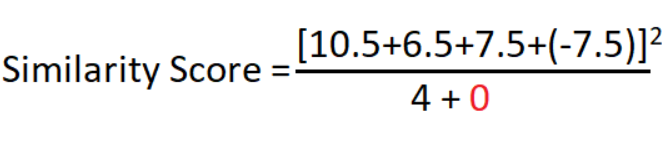The result is: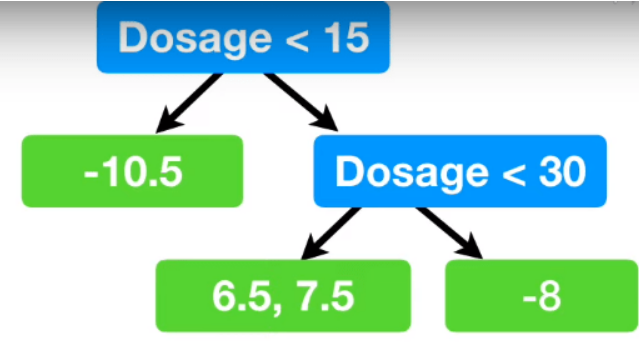## Finally

The machine learning field presents various new and improved technological applications, including XGBoost. Nevertheless, it remains a viable framework concerning prediction accuracy, adaptability, explainability, and practicality.

Due to the parallel and distributed processing it possesses, XGBoost is a quicker method in comparison to other methods. In addition, XGBoost was created with careful regard for both system efficiency and boosting machine learning techniques.

If you want to learn more about XGBoost and other machine learning algorithms, you can visit our Learnbay website or enroll in an Advanced AI and ML program. We'll provide you with the best mentors teaching the latest technologies in the data science field.